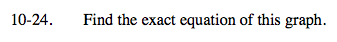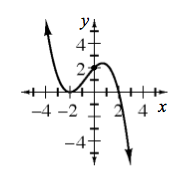### Home > A2C > Chapter Ch10 > Lesson 10.1.1 > Problem10-24

10-24.Determine the roots of the equation.

There are three real roots, one at x = 2 and two at x = −2.

Create a polynomial expressionfrom the roots.

y = a(x + 2)2(x − 2)

Use the y-intercept to solve for a.

(2) = a((0) + 2)2((0) − 2)

$\textit{y}=-\frac{1}{4}(x+2)^{2}(x-2)$